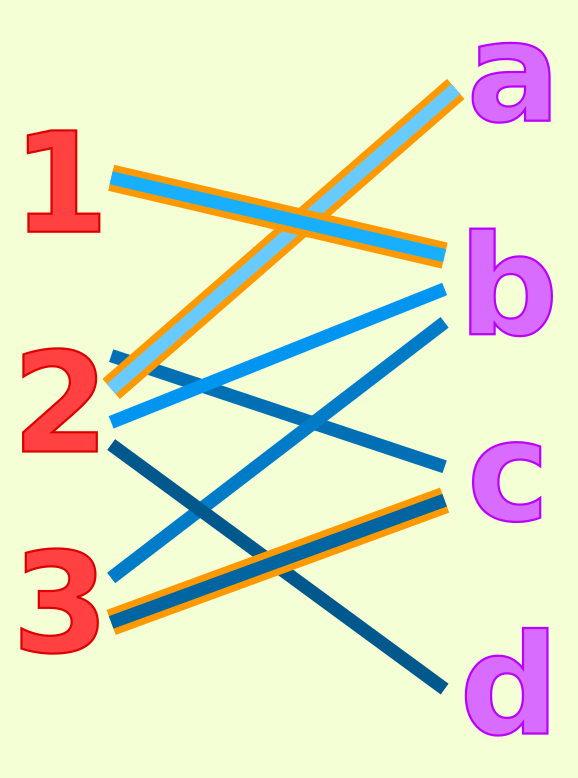# Visual explanation of a Google Codejam 2017 question

This is the last question from the Google Codejam 2017 qualification round.

## Question

How can we place modelson the square stage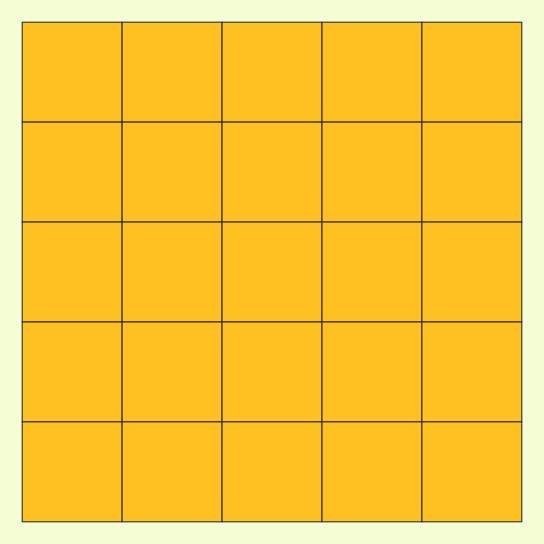to get as many points as possible? The rules are

• If two models on the same row or column, at least one is a +.
• If two models on the same diagonal, at least one is a X.Some models are already placed.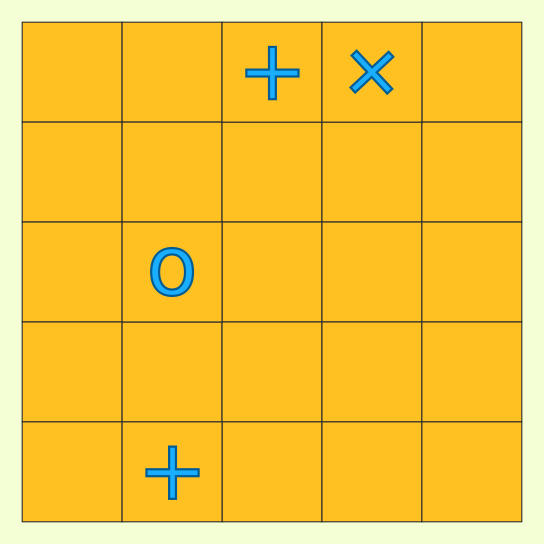We can only change a + or X to an O or place new models on the stage.

## Separation

From the rules, all rows look like this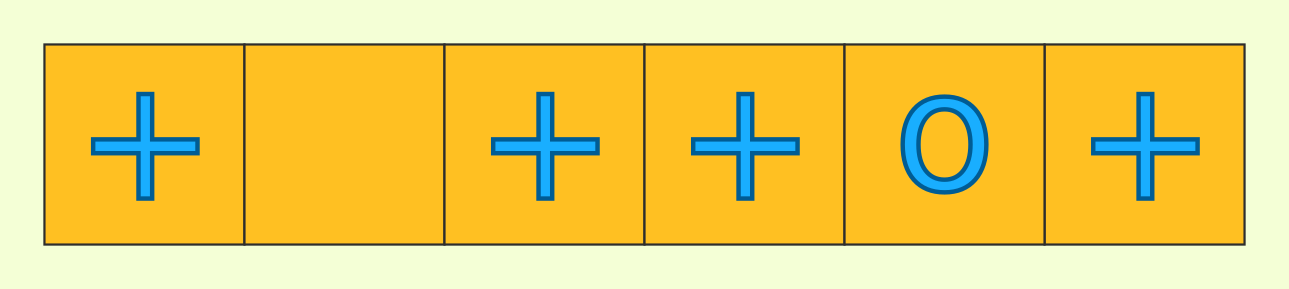where at most one model is not a +. Let's just record where the non-+s are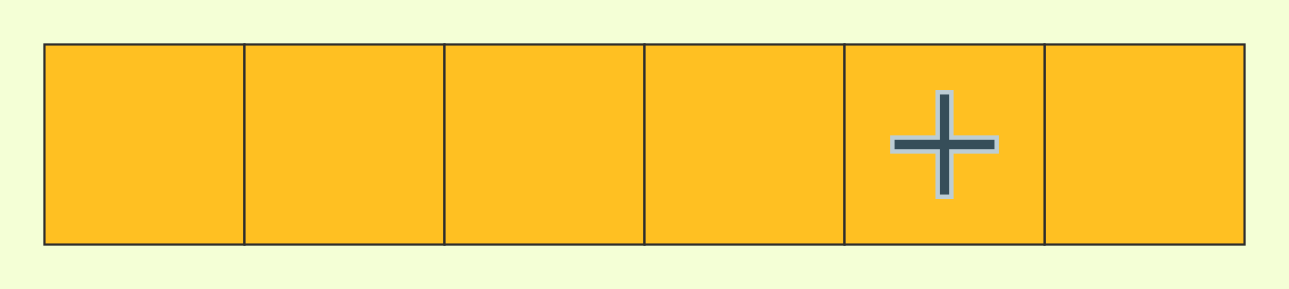The rule is now: at most one non-+ per row and column.

So we can convert a grid (stage) into one grid of non-+ and one grid of non-x.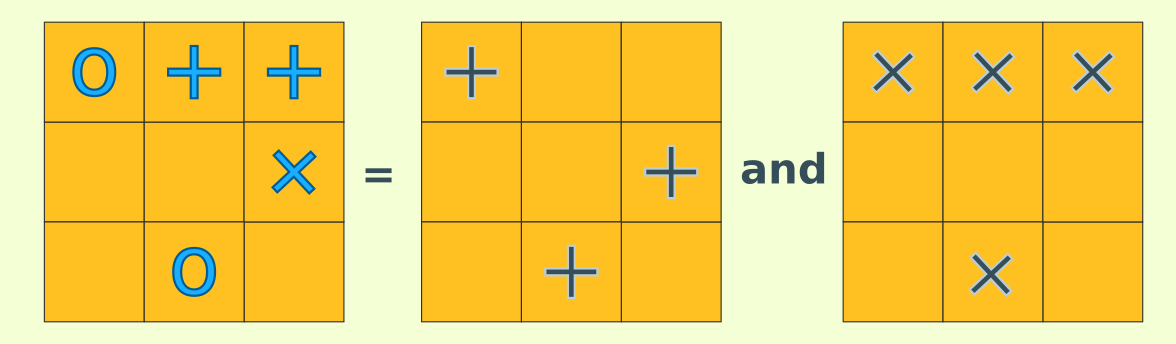using these rules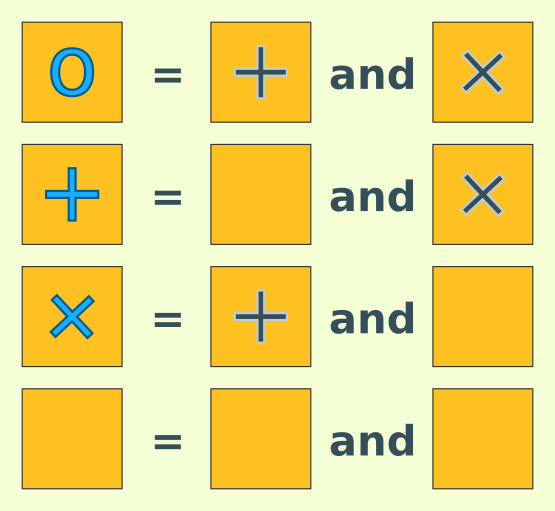and the points match up if each non-+ and non-x is worth 1 style point.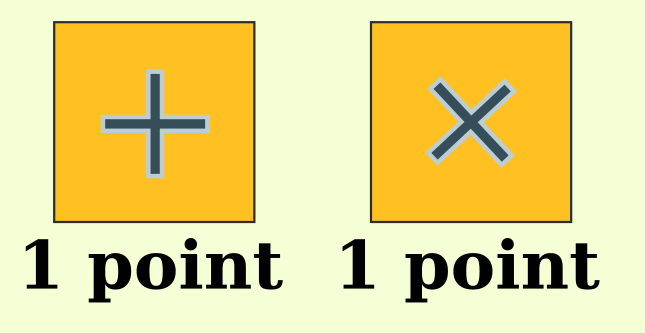The already placed models translate to already placed non-+ and non-x.

This lets us place non-+s and non-xs separately.

## +

non-+ is the easier of the two. A preplaced non-+ means we can't put more non-+ in that row or column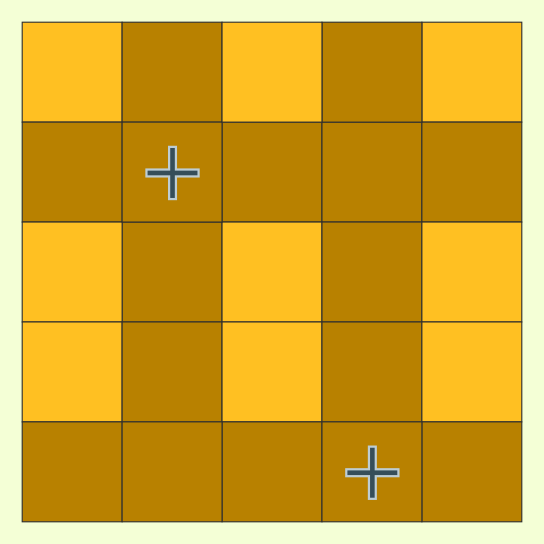so we might as well remove them and get a smaller square. How can we place as many non-+ as possible?Along the diagonal is one way. This places as many non-+ as we have rows (or columns) so we can't do better.

## x

non-x placement is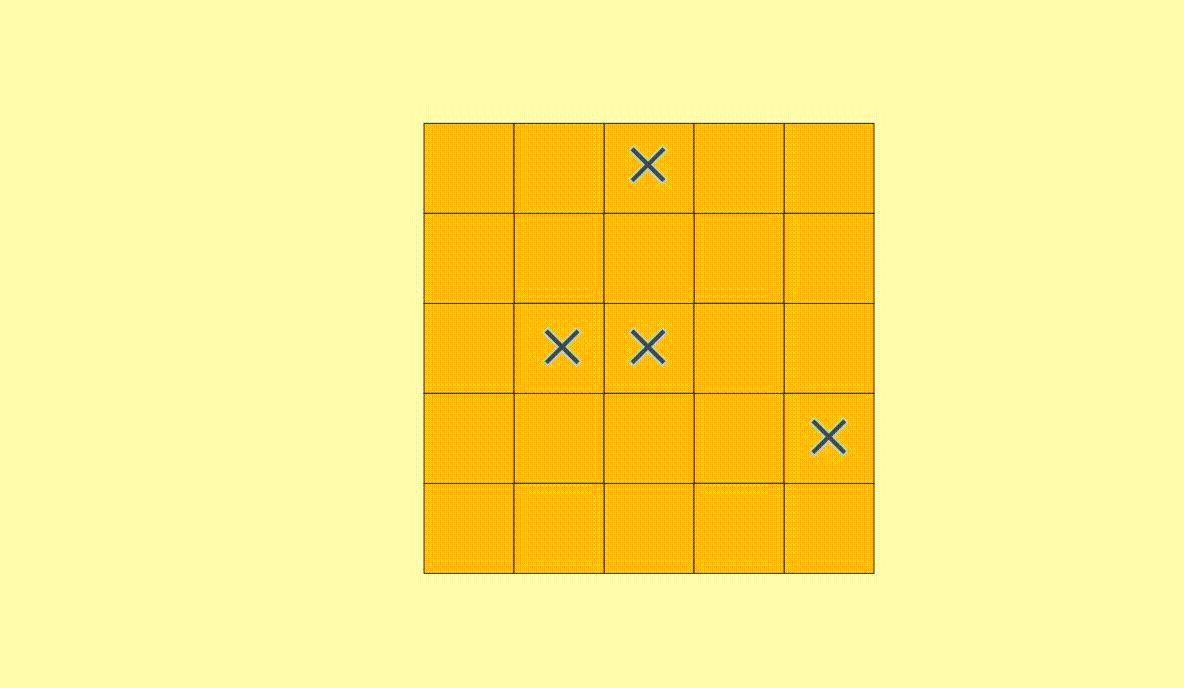non-+ placement rotated by 45 degrees. But now the stage isn't a square anymore. How can be place a maximum number of non-+ here?

This looks like some kind of set packing where the squares are the elements and the rows and columns are the subsets. Since each square appears in exactly two subsets,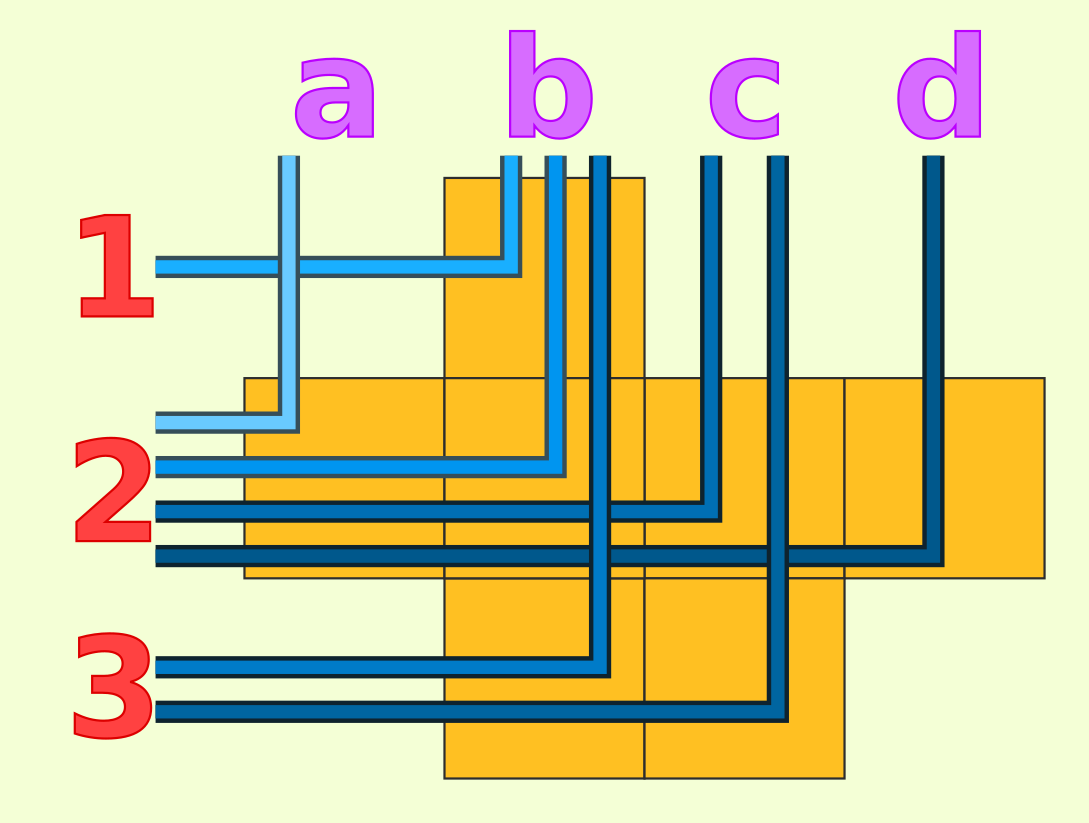set packing here is actually maximum bipartite matching and we can use any of the existing algorithm for that.